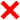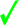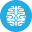# PHP Language | 10 Minute‐Test 5

Instruction

• Total number of questions : 10.
• Time alloted : 10 minutes.
• Each question carry 1 mark.
• No Negative marks
• DO NOT refresh the page.
• All the best :-).

1.

Which version of PHP introduced Try/catch Exception?

A.
 PHP 4B.
 PHP 5C.
 PHP 5.3D.
 PHP 6Correct Answer: Option B

Explanation:

Version 5 added support for Exception Handling.

2.

What will be the output of the following PHP code?

<?php

\$score = 1234;

\$scoreboard = (array) \$score;

echo \$scoreboard;

?>

A.
 1B.
 ErrorC.
 1234D.
 2Correct Answer: Option C

Explanation:

The (array) is a cast operator which is used for converting values from other data types to array.

3.

What will be the output of the following PHP code?

<?php

\$team = "arsenal";

switch (\$team) {

case "manu":

echo "I love man u";

case "arsenal":

echo "I love arsenal";

case "manc":

echo "I love manc"; }

?>

A.
 I love arsenalB.
 ErrorC.
 I love arsenalI love mancD.
 I love arsenalI love mancI love manuCorrect Answer: Option C

Explanation:

If a break statement isn’t present, all subsequent case blocks will execute until a break statement is located.

4.

Which of the following are valid function names?
i) function()
ii) €()
iii) .function()
iv) \$function()

A.
 Only ii)B.
 None of the mentioned.C.
 All of the mentioned.D.
 iii) and iv)Correct Answer: Option A

Explanation:

Except a) others are invalid names. According to the specified regular expression ([a-zA-Z_\x7f-\xff][a-zA-Z0-9_\x7f-\xff]*), a function name like this one is valid.

5.

Which one of the following functions can be used to compress a string?

A.
 zip_compress()B.
 zip()C.
 compress()D.
 gzcompress()Correct Answer: Option D

Explanation:

We will be able to achieve almost 50% size reduction using this function. The gzuncompress() function is used to uncompress the string.

6.

Which in-built function will add a value to the end of an array?

A.
 array_unshift()B.
 into_array()C.
 inend_array()D.
 array_push()Correct Answer: Option D

Explanation:

array_push adds a value to the end of an array, returning the total count of elementsin the array after the new value has been added.

7.

What will be the output of the following PHP code ?

<?php

\$a = array("a" => "Jaguar", "b" => "Land Rover", "c" => "Audi", "d" => "Maseratti");

echo array_search("Audi", \$a);

?>

A.
 aB.
 bC.
 cD.
 dCorrect Answer: Option C

Explanation:

The array_search() function searches for the element and returns the key of that element.

8.

What will be the output of the following PHP code?

<?php

\$a = array("A", "Cat", "Dog", "A", "Dog");

print_r(array_count_values(\$a));

?>

A.
 Array ( [A] => 2 [Cat] => 1 [Dog] => 2 )B.
 Array ( [A] => 2 [Cat] => 2 [Dog] => 1 )C.
 Array ( [A] => 1 [Cat] => 1 [Dog] => 2 )D.
 Array ( [A] => 2 [Cat] => 1 [Dog] => 1)Correct Answer: Option A

Explanation:

The array_count_values() function counts all the values of an array.

9.

Say in the above question you need to get the array sorted in the manner we humans would have done it i.e picture1 then picture2 etc.. Which of the following function should be used?

A.
 dsort()B.
 casesort()C.
 natcasesort()D.
 naturalsort()Correct Answer: Option C

Explanation:

None.

10.

Which one of the following can be used to instantiate an object in PHP assuming class name to be Foo?

A.
 \$obj = new \$foo;B.
 \$obj = new foo;C.
 \$obj = new foo ();D.
 obj = new foo ();Correct Answer: Option C

Explanation:

None.

Try More: Boost your Knowledge
#####Test 27

Submit your test now to view the Results and Statistics with answer explanation.